# All about Gear Effect - 2

Dave Tutelman  --  February 19, 2009

## Vertical gear effect

OK, we have a handle on horizontal gear effect. Now let's look at vertical gear effect, which increases or decreases the backspin a driver puts on the ball. This involves rotation around the red axes in Figure 1-2, caused by impact above or below the center of gravity. The only thing that is different is the moment of inertia; it is Iv instead of Ih. Well, we should probably change the horizontal miss distance x to a vertical miss y. So equation 2, adapted for vertical gear effect, becomes:
s
 58,830 Vb C y Iv
(Equation 3)
That presents a problem. Ih is widely discussed and is sometimes published, so we have a pretty good idea of its magnitude. Iv is not. How do we go about estimating a good value for Iv? Unfortunately, I'm not set up with a Finite Element Analysis program. Every shop that does actual clubhead design uses such programs the way a carpenter uses a Skilsaw. It computes as you design, keeping track of total mass, moment of inertia, stresses, flexes, etc. But I don't have that tool, and I'm not going to compute by hand the MOI of a complex shape like a driver head.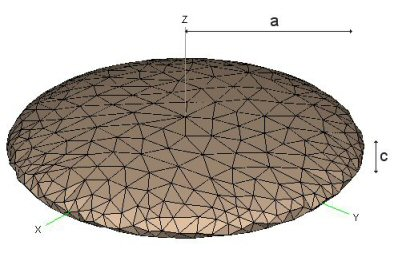Figure 2-1
(From the FEKO web site)
So we'll have to find some way to approximate it. For a first try, let's assume the clubhead is similar to an oblate spheroid, shown in Figure 2-1. An oblate spheroid is a sphere that has been flattened; one "radius" (c in the figure) is smaller than the other two (a in the figure). If a portion of one edge were chopped off to form a clubface, this would be pretty similar to a driver head. In fact, measuring a driver head shows more similarity than you would think just by looking. Let's try an oblate spheroid 4.6" by 2.5". The 4.6" approximates the width and depth of a driver head, the 2.5" approximates it's maximum height, and the total volume is 454cc -- very close to the 460cc legal limit that everybody builds to today.

It is possible to compute the Ih and Iv for an oblate spheroid. Assuming the spheroid is not solid, but rather a shell of uniform thickness, the equations are:
Ih  =  2/3  m a2

Iv  =  1/3  m (a2 + c2)
Using a typical driver mass of 200g, the computed moments of inertia are:
Ih  =  4550 gram-cm2
Iv  =  2950 gram-cm2
This is pretty good. The computed Ih is very close to the average of the moments of inertia we got from the inpakuto.com web site (4575).

But, before I injure myself patting myself on the back, I better note that it is well below the norm for the values in the Golfsmith catalog. Moreover, it does not show much promise of getting near the legal limit set by the Rules. Here is my explanation why the formula should give realistic but low-end values of MOI.

Until quite recently, 460cc heads had significantly higher moments of inertia than previous, smaller heads. So designers were happy to sell them as improvements. A few years ago, there was competitive parity at that point -- 4200 to 4500 g-cm2 -- so designers looked for new ways to increase MOI. They did it by abandoning the uniform thin shell (the assumption in our oblate spheroid model), and lightening some parts of the shell so they could add weight elsewhere and increase the MOI. (In the business, this is called "discretionary weight": weight that is not necessary for structural integrity, so it can be moved to affect things like MOI or CG position.)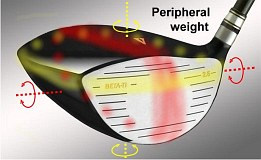Figure 2-2
Using discretionary weight produces a higher Ih than our model accounts for. But what does this do to Iv? Figure 2-2 shows:
• Where you want to add weight to increase Ih (the airbrushed yellow band around the periphery),
• And where weight will increase Iv (the red band around the top and bottom).
Moving weight to increase Ih does not necessarily increase (nor decrease) Iv.

 What designers do to increase Ih What that does to Iv Move weight to the rear of the clubhead. Same increase in moment of inertia. Increase the depth of the clubhead. Same increase in moment of inertia. Move weight to heel and toe. No effect on Iv. Remove weight from crown to obtain discretionary weight for elsewhere. Reduces Iv. Face and sole are hard to take weight from; weight is needed there for structural reasons. And adding weight there does little for Ih, so that is not a candidate. No effect on Iv. Make the head wider (requiring it to be flatter to stay at 460cc). Reduces Iv.

There are two distinctly different possibilities of the best way to estimate  Iv. Without actually measuring heads -- which I am not equipped to do, and such measurements are not widely published -- it is hard to tell which of the two views of Iv is closer to fact. FWIW, here they are:
1. The changes in the above table that increase Ih tend to be somewhere between neutral and a reduction in Iv. So, while the oblate spheroid model shows Iv to be about 2/3 of Ih, it is probably less than that for driver heads with Ih of 5000 g-cm2 or more. I am just guessing, but I would use a value of about half Ih for a head that pushes the USGA limit of  5900 g-cm2. Note that half of 5900 is 2950 -- exactly the same Iv that we computed for the oblate spheroid. Coincidence or not? I don't know. In any event, it looks like somewhere around 3000 g-cm2 is a reasonable value of Iv to use for our sample calculations.
2. Let us remember that the ratio of C to Ih is remarkably constant over a lot of drivers with a variety of moments of inertia. That suggests that the prevalent tool used by designers to boost MOI involves moving weight rearward. That is one of the few items in the table above that increases Iv as it increases Ih. So perhaps the ratio of C to Iv is similarly constant. If so, the ratio runs at about 3/2 that of C to Ih, making vertical spin computable as 1.5*16.4Vby.
I am going to use #2 to compute vertical gear effect, not because I think it is the more correct way (I really don't know), but because it is easier. Besides, if I start with 3000g-cm2 as the moment of inertia, I will have to pick a CG depth, which we know varies a lot from driver to driver. So we will go with:
s  =  25 Vb y            (Equation 3a)
Once again, let's run some real numbers as a sanity test, and compare them with any data that might be around. We will start with our old standby of 150mph ball speed and 11º of loft. We will assume a face roll of 12" radius, same as the bulge. A center-face hit (y=0) will give a distance of 241 yards, as we expected.

Let's move up on the face as high as we dare without losing COR. I'd estimate that to be about 5/8", based on measuring the same drivers I did before. Equation 3a becomes
s  =  25 Vb y  =  25 * 150 * .625  =  2344rpm
That's a lot of spin! And it comes straight off the backspin applied by loft. (Assuming, of course, that the clubhead can rotate freely -- a question we address at the end of this article. But for now let's assume that clubhead rotation is unrestricted.)

How does this square with other investigators of vertical gear effect? Almost everyone I know of has reported much smaller numbers, seldom above 500rpm. Almost everyone. But Dana Upshaw has published data suggesting numbers in this general range. His launch monitor numbers -- 3300rpm difference in spin for a 1.5" difference in height at a ball speed of about 140mph -- are about 63% of the equation's estimate. Not the same, but in the ballpark.

### Spin optimization

Since the contribution of vertical gear effect spin is obviously substantial, we need to ask what it does to the trajectory and the distance. We know that many (perhaps all) golfers will get more total distance with a higher launch angle and less spin than they'd get from a normal center hit on a driver of the "best" loft for their clubhead speed. But that's "and", not "or"; if you increase launch angle at the expense of higher spin, or vice versa, you will lose distance, not gain it. And there are only two ways of independently adjusting launch angle and spin to accomlish this:
• Increase the angle of attack! This increases launch angle without changing the spin.
• Vertical gear effect! This decreses the spin with minimal change of launch angle. (And that minimal change is in the right direction.)
So let's see what happens when we subtract the 2344rpm due to gear effect. At 5/8" above the center, face roll increases the loft to 14º and the launch angle to 12°. So the backspin due to launch angle is 3987rpm, and we have lost 5 yards of distance, back to 235 yards. But that is without factoring in the gear effect!

What happens if we keep the launch angle, but cut the backspin by the amount of gear effect? 3987rpm backspin minus 2344rpm gear effect is 1643rpm net backspin? Whoops! We just lost even more distance. Now we're only 230 yards, down 11 yards from the original 241. We obviously needed some of that spin. But not all of it. A quick optimization with TrajectoWare Drive says a gear effect spin of about 1000rpm would be optimal for that 12° launch. It would cut the spin to just under 3000rpm, add a few yards to the carry, and keep the angle of descent at a reasonable 37°.

There must be some reason we are told to hit high on the face. A few possibilities:
1. We went too far when we went to 5/8".
2. The shaft restricts the rotation of the head, so not as much spin is produced. Less than half of that gear effect spin would be just right.
3. That is where the engineers designed the face for maximum COR. Not likely! It is harder to do than at the center of the clubface, and the engineers' real goal is to make the COR the maximum allowed over the whole clubface. Let's dismiss this out of hand.
We will investigate (b) later in the article. For now, let's look at (a). Here is a table of what happens as we move above the center of the face.

 Height of impact Loft at point of impact Backspin due to loft Topspin due to gear effect Net backspin Distance (yards) Angle of descent Center 11° 3135 rpm 0 3135 rpm 240.5 34° 0.2" above 12° 3416 rpm 750 rpm 2666 rpm 239.3 32° 0.4" above 12.9° 3668 rpm 1500 rpm 2168 rpm 235.3 30° 0.6" above 13.9° 3947 rpm 2250 rpm 1697 rpm 230.0 28°

Conclusions:
• A lot of topspin is generated (actually, a lot of backspin is eliminated). It is too much gear effect to increase the carry for the increased launch angle. The good news is the reduced angle of descent, which will increase the roll after landing.
• We need to see what is behind "door b" -- the restriction of clubhead rotation by the stiffness of the shaft tip. We'll look at that later.
Or maybe there is yet another explanation!
It has been said that god is in the details. There is one detail we have been ignoring.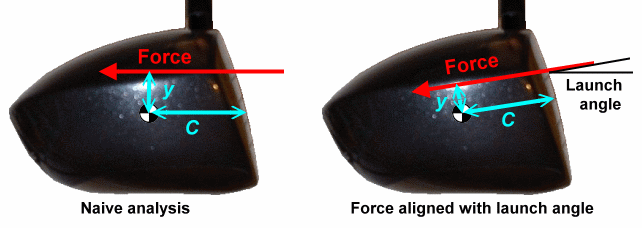Figure 2-3

So far, we have treated the force as being in the direction of the clubhead's movement, as shown in the left image of Figure 2-3. Let's remember that the force is Newton's "equal and opposite reaction" to the departure of the golf ball. A more accurate picture would have the force exactly opposite the departure direction of the ball -- the launch angle -- as shown in the right image of Figure 2-3. This will make a very small difference in C and a much larger difference in y, both in such a direction as to reduce the gear effect. Specifically:
C  =  D cos a

y  =  H - D sin a
Where
• a = launch angle
• D = depth of CG from the center of the face (what we had been calling C until now.)
• H = height of impact above the center of the face (what we had been calling y until now.)
That is not going to make much difference in C (only about 2%, for launch angles below 12°), but it will make a big difference in y. Let's re-do the table above, with this more accurate picture. (We will use D=1.3", consistent with the driver data from inpakuto.com.) Of course, we are going to have to include the launch angle and the actual value of y. We won't bother re-computing C, as the difference will be minimal, way less than any precision in our estimate of D.

 Height of impact Loft at point of impact Launch angle Actual y Backspin due to loft Topspin due to gear effect Net backspin Distance (yards) Angle of descent Center 11° 9.7° -0.22" 3135 rpm -825 rpm 3960 rpm 237.2 41° 0.2" above 12° 10.5° -0.04" 3416 rpm -150 rpm 3566 rpm 241.6 39° 0.4" above 12.9° 11.2° 0.15" 3668 rpm 563 rpm 3105 rpm 244.5 37° 0.6" above 13.9° 12° 0.33" 3947 rpm 1238 rpm 2709 rpm 245.7 35° 0.8" above 14.8° 12.7° 0.51" 4198 rpm 1913 rpm 2285 rpm 244.9 34°

This is more like it! This is what we should have expected. We can wring quite a few extra yards of carry out of a strike 1/2" to 3/4" above the center of the clubface -- and get a bonus of more roll after landing (a consequence of the lower angle of descent).
A few points to carry away from this table:
• A center strike will create vertical gear effect to increase the backspin. That is because the force passes below the CG and rotates the face downward.
• In fact, you have to strike almost 1/4" above center face just to be gear-effect neutral -- no spin due to gear effect. You need that just to get the "nominal" distance out of the driver.
• The topspin due to vertical gear effect looks much more reasonable here. It is still higher than most estimates, but not by nearly as much as before. But...
• If we looked at the consequence of a low hit, we see oodles of gear effect backspin. If we go between 3/4" high and 3/4" low (a total of 1.5") we still see the same spin difference of  5600rpm. But it is biased more towards backspin, since the spin-neutral point is almost 1/4" above the center of the face.

The conclusion from this is that vertical gear effect is a very good reason to try to hit your driver high on the clubface.

Before we leave the subject, I'd like to point out that the real gains will probably be smaller than those in the table. The table was based on a ball speed of 150mph for all the rows. But there are small losses of ball speed as impact moves up the face, due to:
• As we can see in Figure 1-3, the sideways velocity of the face (which creates the gear effect) is accompanied by a backwards velocity. This is essentially a loss of clubhead speed. For a strike 0.6" above center, this is a loss of 1.5%. (But that is not a loss compared with a center strike, which also has a gear effect loss of about 1%. The lossless strike occurs where y=0, about 0.22" above center.)
• There may be some falloff of COR away from the center of the face. How much? That depends on the clubhead designer, and how well it was designed to keep the maximum COR over as much of the face as possible.

## Reality check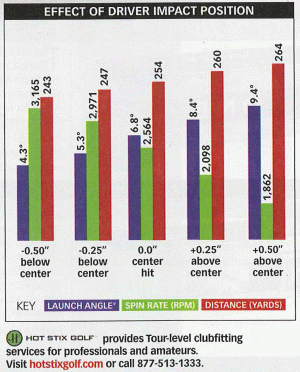Figure 2-4
(Hotstix and Golf magazine)
As we did with the horizontal gear effect, we ought to check the mathematical model against real-world numbers. I was unable to come up with any real data to check against the model when I wrote the article. During subsequent discussion on the TWGT forum, Darryl Green pointed to some recently-published data. There was an article in the Feb 2009 issue of Golf Magazine, disclosing a very useful set of data taken by Hotstix.

They set up a robot to hit a driver from various tee heights, resulting in different impact heights on the clubface. They used a driver that looks like it might be a TaylorMade model, but did not identify it. They described it as a 460cc driver with nominally a 9.5° loft. All the test data was taken at a clubhead speed of 100mph. They measured hits on-center, a quarter inch above and below center, and a half inch above and below center. The data they published for each of those positions were:
• Launch angle in tenths of a degree.
• Backspin in rpm.
• Distance in yards. From the numbers, we can safely assume that was total distance, not just carry distance.

This is sufficient information to give the model a workout. In fact, we don't even need the distance data. The important thing is to determine if a 100mph clubhead speed and the given face heights and launch angles, when fed to the model, gives the same backspin that Hotstix measured.

Here's a table of how the model fared.

 Input from Hotstix article From TrajectoWare Drive From model H Launch angle Measured total backspin Loft at impact Backspin due to loft Ball speed y Gear effect backspin Computed total backspin Error (rpm) -0.50" 4.3° 3165 4.6 1283 148 -0.60" 2210 3494 10.4% -0.25" 5.3° 2971 5.8 1617 148 -0.37" 1369 2986 0.5% 0 6.8° 2564 7.5 2088 148 -0.15" 570 2657 3.6% +0.25" 8.4° 2098 9.4 2613 147 +0.06" -221 2392 14.0% +0.5" 9.4° 1862 10.6 2943 146 +0.29" -1050 1893 1.7%

And here's where each column in the table comes from:
• Green columns are raw data, numbers copied from Hotstix article.
• For the blue columns, I cranked 100mph ball speed into TrajectoWare Drive. Then I changed the loft until I got the same launch angle as the data. The blue columns record that loft, as well as ball speed and spin.
• The yellow columns are the predictions by the mathematical model
• The value of y was computed from  y  =  H - D sin a. I assumed a 1.3" CG depth for the driver, and used the measured H and launch angle.
• The gear effect spin was computed from equation 3a: s  =  25 Vb y. (For this table, I used the convention that backspin is positive and topspin is negative.)
• The computed total backspin is the sum of the backspin due to loft and the gear effect backspin. This is the number to be compared with the measured total backspin in the Hotstix data.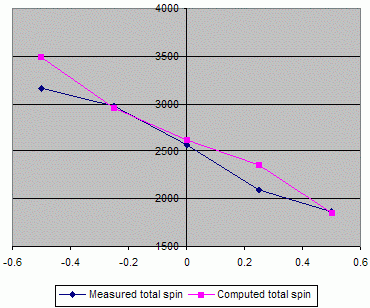Figure 2-5
We can look at the comparison numerically in the table, and also look at it graphically in Figure 2-5.

Given the coarseness of the assumptions, there is a remarkably good match between data and model. Three of the five data points are within 4%, which is scary-good considering how we approximated the MOI and CG depth. The other two points are off by only 10% and 14%. And they are not clustered together at one end of the data, which might have suggested a trend that the model was missing.

Bottom line: the data strongly suggests the model is close enough to reality to be very useful.

### Improving the match

Given how good the match is, I should probably just give it a rest and declare success. But let's see if sharpening our assumptions could make the match even better.  What parts of our model should we allow to change, and what parts are just Newtonian physics and obviously correct? Let's look at equation 3, the basis of the model:
• Gear effect spin is proportional to ball speed. That's basic, so leave it alone.
• Gear effect spin is proportional to y, the distance between the force of impact and the CG of the clubhead. Again, that is basic; it is the moment arm for the torque on the head.
• Gear effect is proportional to the center of gravity depth C. Fairly basic, but the value we used for C was based on some rough assumptions.
• Gear effect is inverstely proportional to the moment of inertia Iv. Totally basic, but again the value for Iv was based on rough assumptions.
The most suspect thing about the model (as expressed in equation 3a) is the assumption-heavy way we approximated the vertical moment of inertia Iv and the depth of the center of gravity C. If we play around with these values and watch the error column, we can try to find values that minimize the error. I set up a spreadsheet and did that. Specifically, I played with the coefficient 25 in equation 3a and values for C other than our 1.3".

With a CG depth of 1.1", all the errors are below 10%. No amount of fooling with Iv did much to reduce the error. That is a plausible set of values. The CG depth is at the low end of the data we used earlier (from the Alba magazine study), but still within range. Now, remember that we don't know much about the specific driver Hotstix used for the test. If it had a relatively short CG depth, then the model should be using something like 1.1" for C.

Could it be even better? Not with the raw data we have. I think we are bumping up against the limits of measurement error at this point. There are "wiggles" when we plot the data -- spin vs face height and launch angle vs face height -- that suggest inaccuracies in the measurements. To test this, I smoothed the data using a best-fit second-order polynomial for spin and launch angle. (I didn't want to force a best-fit straight line, in case the real underlying effect is nonlinear. Including a second-order term allows curvature but not enough wiggling to be able to fit any five data points arbitrarily.) The results are shown in Figure 2-6.Figure 2-6
The figure shows the actual data points, the best-fit "line", and the equation for that line. (The best-fit lines actually have some second-order curvature, as shown by an x-squared term in the equation. The fact that curvature is hard to see indicates that the data is nearly linear.)

We see that the differences between the data and the line are less than 100rpm for the spin data and less than 0.2 degrees for the launch angle data. If you are familiar with how spin and launch angle are measured, you know that these could easily be measurement error. It is really quite creditable how good the measurements are.

What I did next was re-plot the model's output using the "smoothed" data -- the best-fit curves -- instead of the raw data. Now the model fit even better.

With a CG depth of 1.1" and a coefficient of 25, only one point was over 4% error, and that was 6.6%.

And, if I played more with the model, at 1.0" and 23.5 the errors were all under 3%.

Conclusion: With the match between the model and the data, the model is accurate enough to be very useful.

For the remainder of this article, we will continue with the model using a coefficient of 25, because that is plenty close enough to the data. We will also stay with C=1.3" for the "generic" driver, though we will use the actual C if we know it.